### Examples

 Simple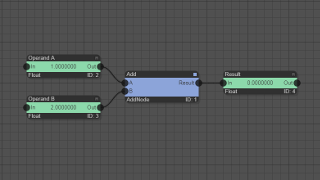Add This very simple example adds two floating point numbers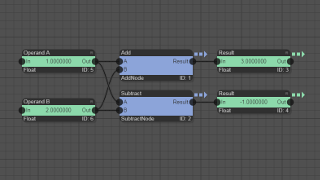AddSub This example adds and subtracts the same two floating point values in one time step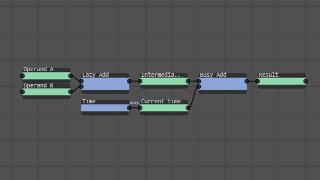Lazy In this example, the current time is used as an operand in an addition. Note, how the first addition in the graph is executed only once (lazy evaluation) whereas the second add is executed in each time step.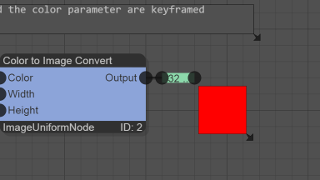Keyframes This is an example for an automated change of public parameters (of type text and color) using keyframes. Vectors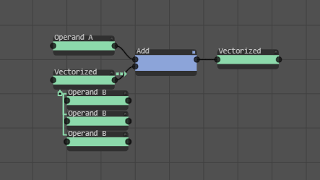VectorAdd Vectorization can simplify a graph because many operations of the same type can be excuted with a single compute node instance. This example is an extention of the simple "Add" example. Now, one of the operands is a vectorized data node of type FloatVector, instead of a simple Float. The addition is computed for each child of the FloatVector and written to the FloatVector children that are generated at the output.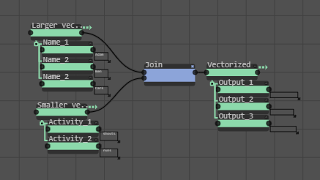DifferentSizes This example demonstrates what happens when a compute node has several vectorized data nodes as an input and these are not of the same size.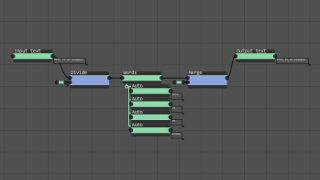DivideMerge This examples divides a text into words using space as a separator. The output of the divide compute node is a vector of words of type [TextVector]. This vector is then merged again into a single text in which the words are separated by commas. Image CompositingRocket In this example, a moving rocket is placed on top of a static background.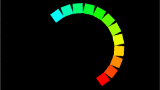FollowShape In this example, several images are moving alone a predefined path (e.g. a circle).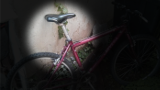Torchlight This example uses a compositing mask to generate a simple torchlight effect.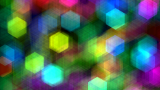Accumulate This example demonstrates the usage of the accumulate node.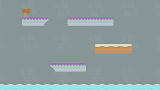Tile Map In this example, it is shown how tiles from a tile map can be composited into a scrollable background image for 2D games.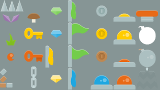Sprite Sheet This example demonstrates how to composite an image from many sprites defined in a sprite sheet.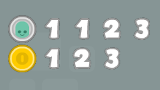Sprite Grid This example demonstrates how to generate a scoreboard for a 2D game using the SpriteGrid node. Image Processing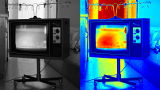PseudoColor This example demonstrates how to convert a grayscale image to a color image using a selectable colormap.EdgeDetection In this example, a Sobel filter is used to perform edge detection.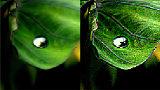Filter3x3 This example shows how to work with your own custom filter kernels for image filtering.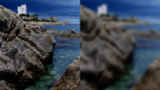Separable This example shows how to perform image filtering using custom separable filter kernels.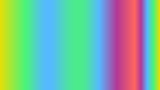Multi-Gradient This example demonstrates how to generate a multi-color gradient image. The individual color transitions are controlled via the editable interpolation function.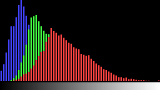Histogram Bars In this example, a histogram of a selected image region is computed and visualized as a bar chart.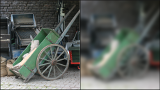Fourier2D This example demonstrates the usage of a 2D Fourier transform and its inverse to perform image filtering.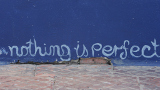BatchProcessing This example shows how to execute several image processing operations on a batch of images.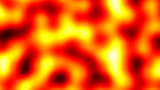PerlinNoise This example shows how to generate 3D Perlin noise. Audio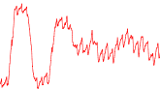Play Audio File This example shows how to load an audio file as a signal and play it.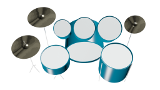Virtual Drums In this example, a virtual drum kit can be triggered with keyboard keys or with mouse clicks.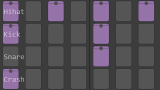Drum Machine Basic In this example, the step sequencer node is used to generate a simple drum machine from four audio samples.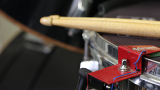Drum Machine Drum machine example with funky drum sounds. Sounds can be changed by creating a new project and uploading own audio samples.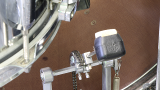Rock Drums Drum machine example with rock drum sounds.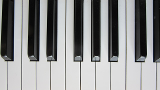Instrument In this example, an instrument node is used to create 72 semitones of a piano from a total of 14 audio samples. The 72 semitones are fed as inputs to a step sequencer node, which plays the beginning of Beethoven's "Für Elise".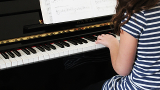Abc Song The tonality node generates notes from the major and minor musical scale for a selected base key. In this example, the classic AbcSong kids song can be interactively changed from major to minor scale or the spacing of notes within the scale can be altered.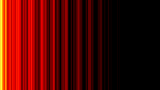Stream Delay This example shows how to route the output of a step sequencer through a delay effect (at output channel 1).Kick Drum In this example, a kick drum sound is synthesized by pitch and amplitude modulation of a sine function.Bass Synth This example demonstrates how to synthesize a simple bass sound by modulating the mixing value of a distorted and undistorted sine function over time. Furthermore, in subsequent steps, pitch and amplitude modulation is applied. VideoMultiMirror This example shows how to apply a multi-mirror effect on a video. Video source: Big Buck Bunny, Blender Foundation, CC-BY Paths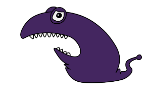MultiplePaths This example shows how to render multiple paths with different styles into an image (and how to export them as an SVG drawing).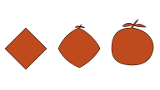PathInterpolation In this example, an interpolation between two paths is demonstrated. 3D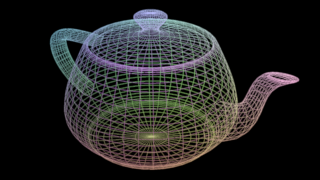LoadMesh This example shows how to load a 3D mesh. Currently, only the Wavefront OBJ format is supported.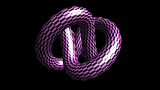BlinnPhong In this example, a 3D mesh is rendered with the Blinn-Phong shading model. Signal Processing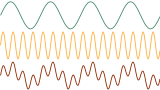Lowpass In this example, two sine waves with a lower and a higher frequency are superimposed by an addition. By using a low-pass and a high-pass filter, it is possible to separate the signals again.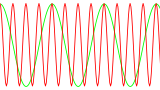DFT In this example, a cosine wave is transformed into the frequency domain by using the discrete Fourier transform (DFT). Afterwards the original signal is recovered with the inverse discrete Fourier transform (IDFT).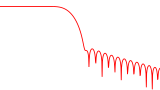Frequency Response This example computes the frequency response of a low-pass filter in decibels. The cut-off frequency of the low-pass filter is changed over time.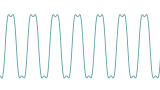Custom Filter This example shows how to apply a custom filter to a signal. Apparent Loops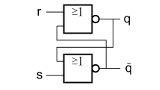FlipFlop This examples shows how to use the DelayedCopy node to create a simulation of a RS flip flop. General information about flip flops can be found on Wikipedia.FollowMouse In this example, two images are composited and the foreground image follows the mouse pointer position. Plugin Node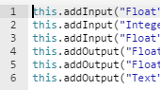Numbers This example shows how to work to with float and integer nodes using the plugin node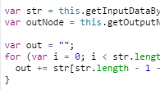ReverseText This examples shows how to reverse a text using the plugin node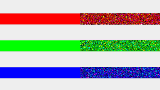RandomColorOffset This examples shows how to work with color nodes using the plugin node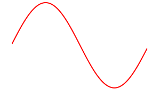Sine This examples shows how to work with a signal node using the plugin node. GLSL ImageShader Plugin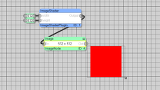AllRed A very simple example for a GLSL image shader plugin that sets all pixels to red.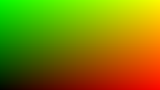TextureCoordinates In this GLSL example, texture coordinates are introduced.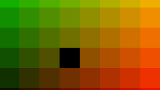OneBlack This GLSL example shows how to convert texture coordinates to pixel coordinates.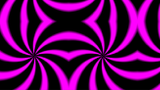TextureParameter This GLSL example shows how to change the texture parameters of input images.MouseAsUniform This example shows how to access the current mouse position from GLSL shader code.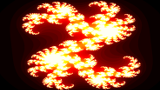JuliaFractal In this example, the Julia set fractal is computed using GLSL code.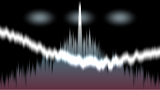AnalyseSound This example shows how to setup a GLSL ImageShader that reacts to sound input. GLSL Shader Plugin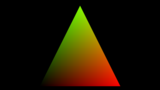FirstShader This example uses a minimalistic shader that renders a mesh with the texture coordinates as colors.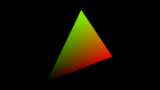ShaderUniform This sample shader introduces a projection and a modelview transformation matrix that are passed as uniform variables.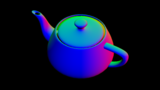NormalTrans In this example, it is shown how mesh normals can transformed with the transposed inverse modelview matrix.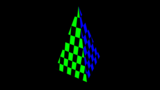ShaderTexture This example shows how to access a 2D texture in the shader.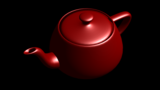ShaderBlinnPhong This shader example computes Blinn-Phong shading with a directional light.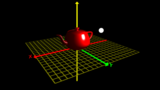ShaderPhongPointLight This shader example computes Blinn-Phong shading with a point light.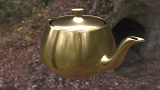ImageBasedLighting This example contains a plugin shader node that performs image-based lighting.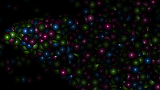ParticleShader This is a basic setup for modifying particles with GLSL shaders. Dependent on the capabilities of the employed GPU, several hundred-thousands of particles can be handled.